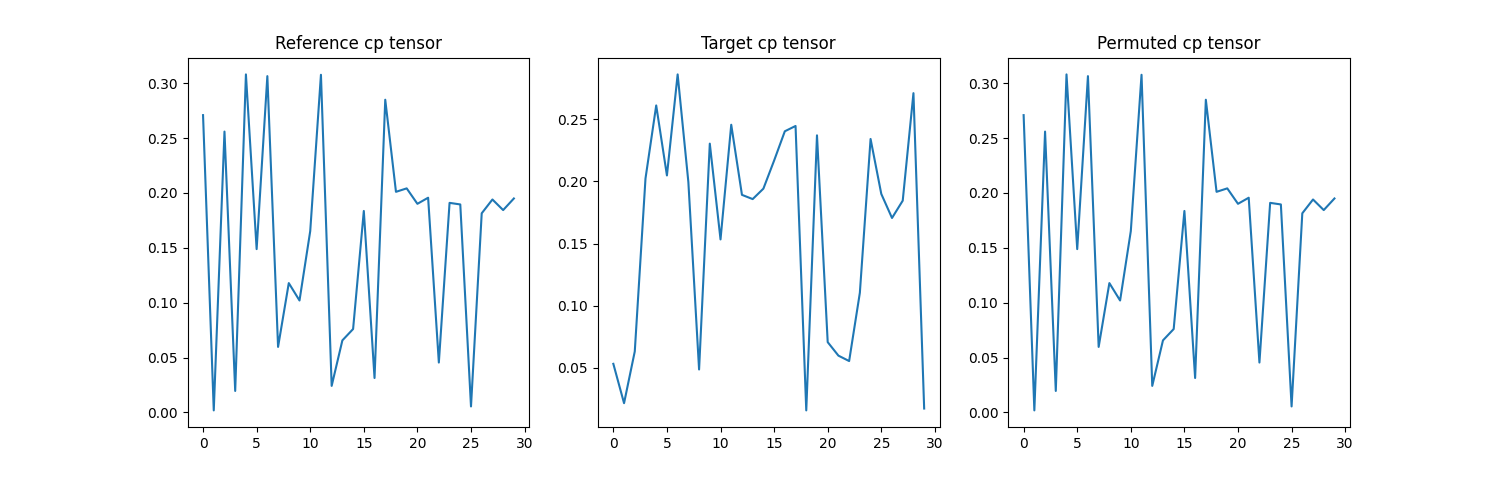# Permuting CP factors

On this page, you will find examples showing how to use tensorly.cp_tensor.cp_permute_factors function.

## Introduction

This function compares factors of a reference cp tensor with factors of another tensor (or list of tensor) in order to match component order. Permutation occurs on the columns of factors, minimizing the cosine distance to reference cp tensor with scipy Linear Sum Assignment method. The permuted tensor (or list of tensors) and list of permutation for each permuted tensors are returned. Tensorly CPTensor should be used as an input to permute their factors and weights simultaneously.

```import tensorly as tl
from tensorly.random import random_cp
from tensorly.cp_tensor import cp_permute_factors
import matplotlib.pyplot as plt
```

## Create synthetic tensor

Here, we create a random tensor, then we permute its factors manually.

```shape = (30, 40, 50)
rank = 4

# one reference cp tensor
cp_tensor_1 = random_cp(shape, rank)

# two target cp tensors
cp_tensor_2 = cp_tensor_1.cp_copy()
cp_tensor_3 = cp_tensor_1.cp_copy()

col_order_1 = [1, 0, 3, 2]
for f in range(3):
cp_tensor_2.factors[f] = cp_tensor_2.factors[f][:, col_order_1]

col_order_2 = [3, 1, 2, 0]
for f in range(3):
cp_tensor_3.factors[f] = cp_tensor_3.factors[f][:, col_order_2]
```

## Permute target CPTensors

Now, we can use these two manipulated CPTensors as inputs to the permutation function. Here, cp_tensor_1 will be used as a reference to permute other CPTensors, which are called target CPTensors. There is no limitation for the number of target CPTensors but there should be only one reference CPTensor. Results will include permuted CPTensors and permutation for each permuted cp tensor. It should be noted that, reference CPTensor won’t be included among the output CPTensors.

```cp_tensors, permutation = cp_permute_factors(cp_tensor_1, [cp_tensor_2, cp_tensor_3])
```

As it is expected, permutation variable stores two lists which are equal to predefined col_order_1 col_order_2 above.

```print(permutation)
```
```[array([1, 0, 3, 2]), array([3, 1, 2, 0])]
```

We can also observe the evolution of the factor columns order by plotting one column before and after permuting.

```fig, axs = plt.subplots(1, 3)
fig.set_size_inches(15, fig.get_figheight(), forward=True)
axs.plot(cp_tensor_1.factors[:, 0].T)
axs.set_title("Reference cp tensor")
axs.plot(cp_tensor_2.factors[:, 0].T)
axs.set_title("Target cp tensor")
axs.plot(cp_tensors.factors[:, 0].T)
axs.set_title("Permuted cp tensor")
``````Text(0.5, 1.0, 'Permuted cp tensor')
```

Total running time of the script: ( 0 minutes 0.168 seconds)

Gallery generated by Sphinx-Gallery